# SPSS tutorials

BASICS DATA ANALYSIS T-TEST ANOVA CHI-SQUARE TEST

# SPSS Mean Centering and Interaction Tool

A sports doctor wants to know if and how training and age relate to body muscle percentage. His data on 243 male patients are in muscle-percent-males.sav, part of which is shown below.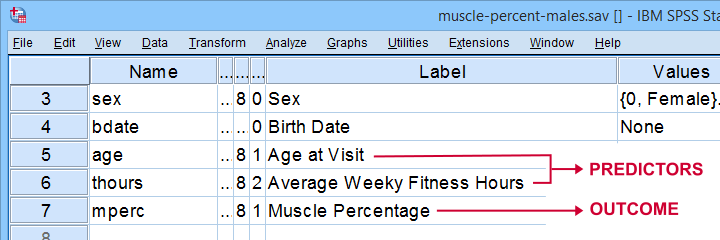## Regression with Moderation Effect

The basic way to go with these data is to run multiple regression with age and training hours as predictors. However, our doctor expects a moderation interaction effect between age and training. Precisely, he believes that the effect of training on muscle percentage diminishes with age. The diagram below illustrates the basic idea.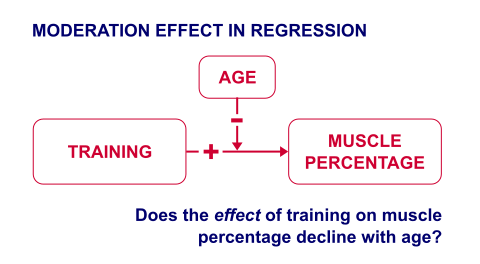The moderation effect can be tested by creating a new variable that represents this interaction effect. We'll do just that in 3 steps:

1. mean center both predictors: subtract the variable means from all individual scores. This results in centered predictors having zero means.
2. compute the interaction predictor as the product of the mean centered predictors;
3. run a multiple regression analysis with 3 predictors: the mean centered predictors and the interaction predictor.

Steps 1 and 2 can be done with basic syntax as covered in How to Mean Center Predictors in SPSS? However, we'll present a simple tool below that does these steps for you.

First off, you need SPSS with the SPSS-Python-Essentials for installing this tool. The tool is downloadable from SPSS_TUTORIALS_MEAN_CENTER.spe.
After downloading it, open SPSS and navigate to ExtensionsInstall local extension bundle as shown below.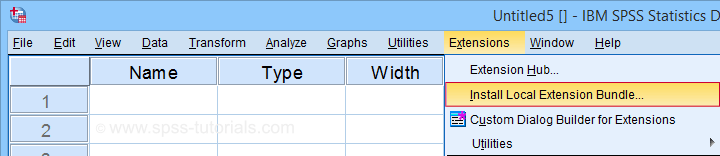For older SPSS versions, try UtilitiesInstall local extension bundle You may need to run SPSS as an administrator (by right-clicking its desktop shortcut) in order to install any tools.

## Using the Mean Centering Tool

First open some data such as muscle-percent-males.sav. After installing the mean centering tool, you'll find it in the Transform menu.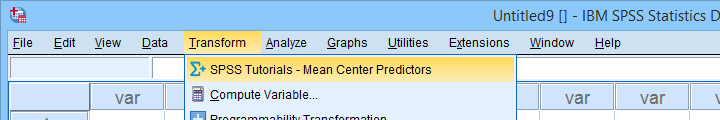This opens a dialog as shown below. Note that string variables don't show up here: these need to be converted to numeric variable before they can be mean centered.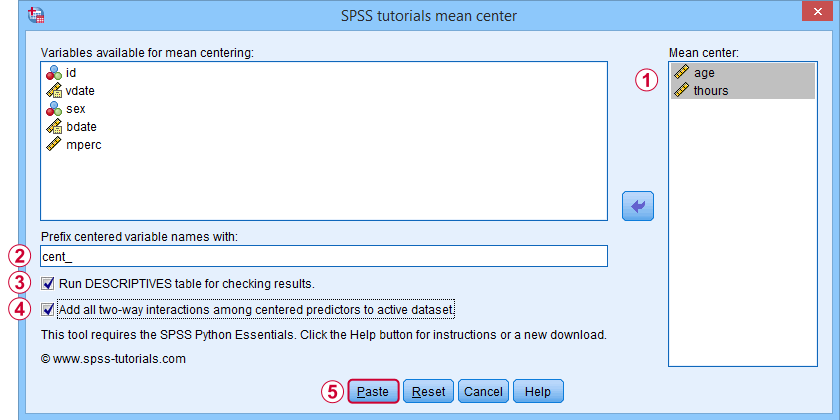Variable names for the the centered predictors consist of a prefix + the original variable names. In this example, mean centered age and thours will be named cent_age and cent_thours.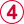Optionally, create new variables holding all 2-way interaction effects among the centered predictors. For 2 predictors, this results in only 1 interaction predictor.Clicking results in the syntax below. Let's run it.

*Mean center 2 variables, compute 1 interaction effect and print a checktable.

SPSS_TUTORIALS_MEAN_CENTER VARIABLES = "age thours"
/OPTIONS PREFIX = cent_ CHECKTABLE INTERACTIONS.

## Mean Centering Tool - Results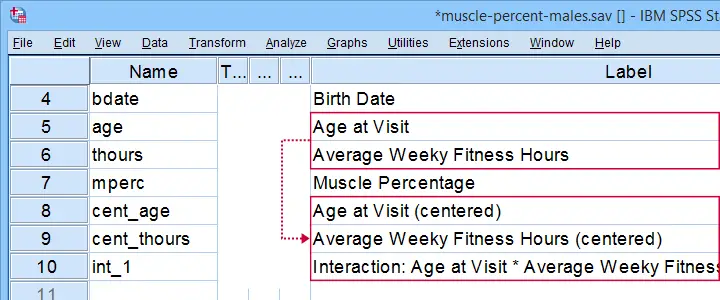In variable view, note that 3 new variables have been created (and labeled). Precisely these 3 variables should be entered as predictors into our regression model.
If a checktable was requested, you'll find a basic Descriptive Statistics table in the output window.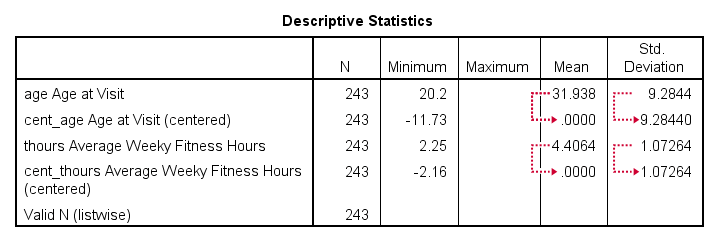Note that the mean centered predictors have exactly zero means. Their standard deviations, however, are left unaltered by the mean centering -which is precisely how this procedure differs from computing z-scores.

Right, so that'll do for our mean centering tool. We'll cover a regression analysis with a moderation interaction effect in 1 or 2 weeks or so.